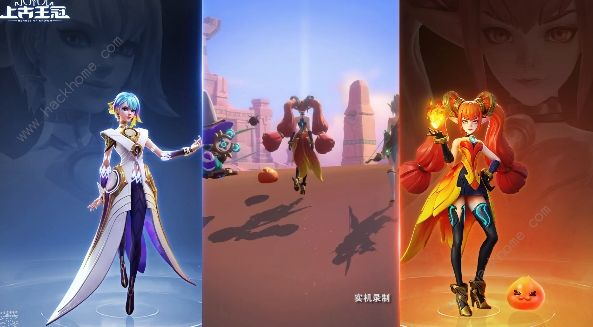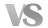55+0+000221650000
4+1+0161241000
3+2+0181332000
3+1+111831100
2+2+1141022100
44+0+011+0201540010
3+1+0151131010
2+2+0181322010
2+1+111821110
33+0+022+0211530020
2+1+02+0171221020
1+1+12+010711120
3+0+01+1191430011
2+1+01+1151121011
1+1+11+1171211111
22+0+033+0221620030
1+1+03+0151111030
2+0+02+1211520021
1+1+02+1141011021
11+0+044+0221510040
3+1191410031
2+2201410022
0055+0302000050
4+1261800041
3+22518000321单色队（50000、00050）理论加成很高，但组建难度很大，如果想要实现这类阵容，会闲置很多其他阵营的卡牌，性价比很低，所以忽略纯色队，即使它们加成很高。

2三色、四色队的实现难度都不高，在这些队形中，加成相对较高的队形编号会被标亮，即：

22010----[(2水2火/2火2风/2风2水)+(1光/1暗)]

30020----[(3水/3火/3风)+(2光/2暗)]

30011----[(3水/3火/3风)+(1光1暗)]

20030----[(2水/2火/2风)+(3光/3暗)]

20021----[(2水/2火/2风)+(2光1暗/1光2暗)]

10022----[(1水/1火/1风)+(2光2暗)]

3五色队（11111）很容易组建，但加成不高，不需要刻意去实现。

50%

050%

0

(您的评论需要经过审核才能显示)